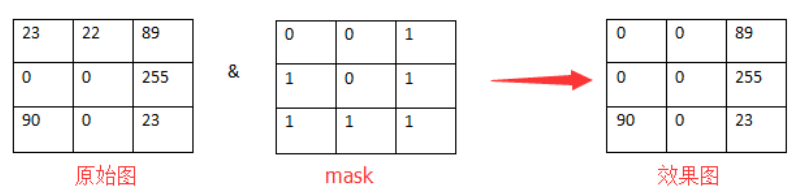2016-12-05 17:27:18 jinxiaonian11 阅读数 66757
• ###### MATLAB图像处理

全面系统的学习MATLAB在图像处理中的应用

19898 人正在学习 去看看 魏伟

①提取感兴趣区,用预先制作的感兴趣区掩模与待处理图像相乘,得到感兴趣区图像,感兴趣区内图像值保持不变,而区外图像值都为0。
②屏蔽作用,用掩模对图像上某些区域作屏蔽,使其不参加处理或不参加处理参数的计算,或仅对屏蔽区作处理或统计。
③结构特征提取,用相似性变量或图像匹配方法检测和提取图像中与掩模相似的结构特征。
④特殊形状图像的制作。

2019-01-04 00:25:59 qq_42887760 阅读数 371
• ###### MATLAB图像处理

全面系统的学习MATLAB在图像处理中的应用

19898 人正在学习 去看看 魏伟

# 1. 获取图像像素指针

• CV_Assert(myImage.depth() == CV_8U);
• Mat.ptr(int i=0) 获取像素矩阵的指针，索引i表示第几行，从0开始计行数。
• 获得当前行指针const uchar* current= myImage.ptr(row );
• 获取当前像素点P(row, col)的像素值 p(row, col) =current[col]

# 2. 像素范围处理saturate_cast

saturate_cast（-100），返回 0。
saturate_cast（288），返回255
saturate_cast（100），返回100

# 3. 掩膜操作实现图像对比度调整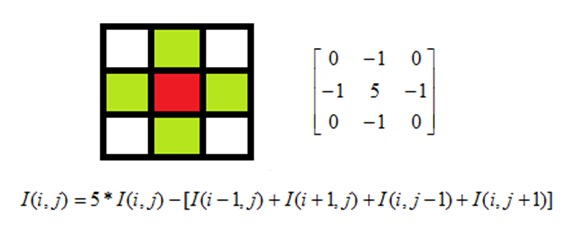• 掩膜矩阵 3*3 在图像矩阵上移动与图像重合，与每一个重合的像素点做掩膜操作，

• 公式：中心点掩膜后的颜色数据 $I(i,j) = 5*I(i,j) - [I(i-1,j)+I(i+1,j)+I(i,j-1)+I(i,j+1)]$

• 这里是3*3的矩阵，所以图像数据的第一行倒数第一行，第一列倒数第一列不做掩膜操作 。其中： i，j 表示像素的位置，第 i 行，第 j 列， I（i，j） 表示每个通道颜色数据。

• 掩膜操作不是矩阵乘法，由公式可以看出

• 该掩膜矩阵的作用： 掩膜操作可以提高图像对比度，对比度提高可以增加图像感官度、锐化，让看起来有点模糊的图像更清晰

###### 程序代码
#include<opencv2/opencv.hpp>
#include<iostream>
#include<math.h>

using namespace cv;

int main(int argc,char** argv){
Mat src,dst;

if(!src.data){
return -1;
}
//CV_Assert(src.depth()==CV_8U);

//掩膜操作
int channels=src.channels();//图像的通道数
int cols=(src.cols)*src.channels();//列数*通道数
int rows=src.rows;//行数

dst=Mat::zeros(src.size(),src.type());//初始化 dst

for(int row=1 ; row<rows-1 ; row++){
const uchar* previous = src.ptr<uchar>(row - 1);//上一行
const uchar* current=src.ptr<uchar>(row);//当前行
const uchar* next =src.ptr<uchar>(row + 1);//下一行

uchar* output=dst.ptr<uchar>(row);
for(int col=1*channels ; col<cols-1*channels ; col++){
//掩膜操作：I(i,j) = 5*I(i,j) - [I(i-1,j)+I(i+1,j)+I(i,j-1)+I(i,j+1)]
output[col] =saturate_cast<uchar>( 5 * current[col] - (previous[col] + next[col] + current[col + channels] + current[col + channels]));
}

}

namedWindow("output1",CV_WINDOW_AUTOSIZE);
imshow("output1",src);

namedWindow("output2",CV_WINDOW_AUTOSIZE);
imshow("output2",dst);

waitKey(0);

return 0;
}

###### 运行效果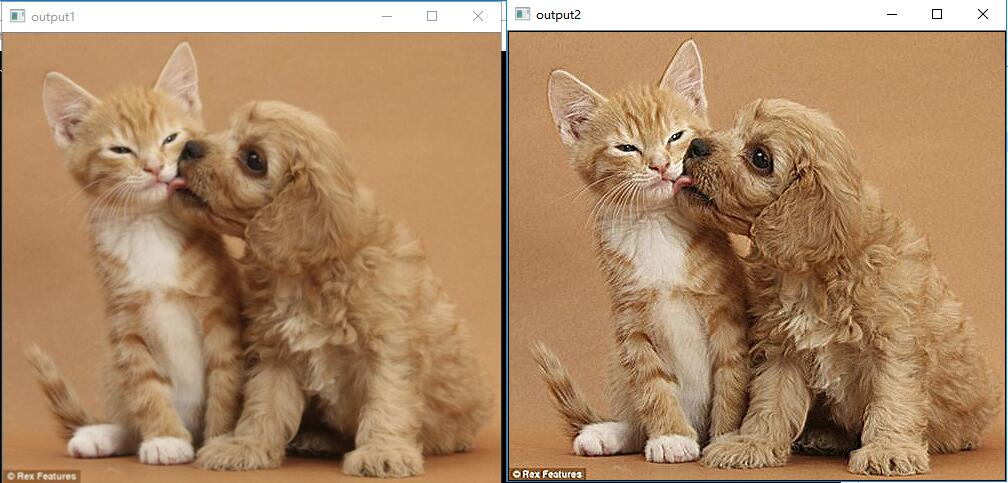（即：将32行中output[col] =saturate_cast( 5 * current[col] - (previous[col] + next[col] + current[col + channels] + current[col + channels]));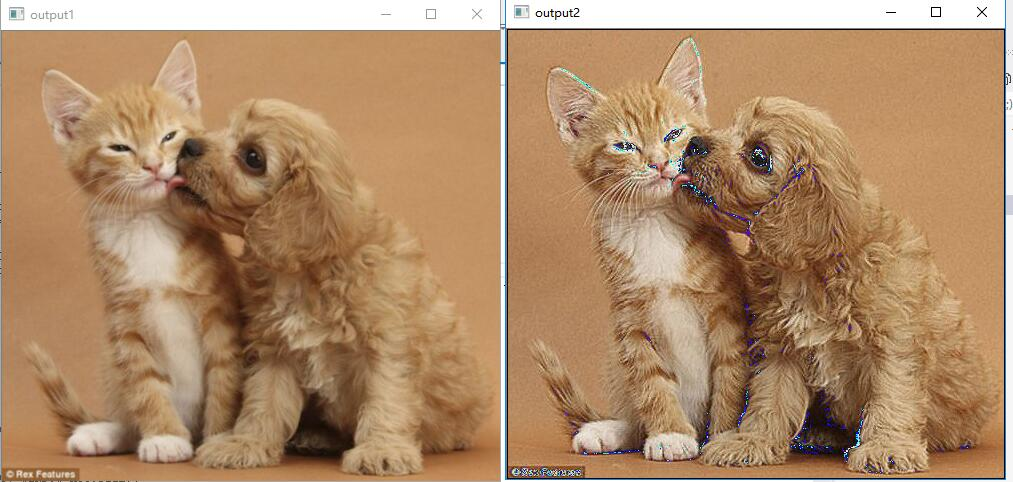# 4. 函数调用filter2D功能

• 定义掩膜：Mat kernel = (Mat_(3,3) << 0, -1, 0, -1, 5, -1, 0, -1, 0);
• filter2D( src, dst, src.depth(), kernel ); 其中src与dst是Mat类型变量、src.depth表示位图深度，有32、24、8等。
###### 代码
#include<opencv2/opencv.hpp>
#include<iostream>
#include<math.h>

using namespace cv;

int main(int argc,char** argv){
Mat src,dst;

if(!src.data){
return -1;
}

Mat kernel=(Mat_<char>(3,3)<< 0, -1, 0, -1, 5, -1 , 0, -1, 0);
filter2D(src,dst,src.depth(),kernel);
//filter2D(src,dst,-1,kernel);

namedWindow("output1",CV_WINDOW_AUTOSIZE);
imshow("output1",src);

namedWindow("output2",CV_WINDOW_AUTOSIZE);
imshow("output2",dst);

waitKey(0);

return 0;
}

###### 运行效果（与上述代码效果一致）：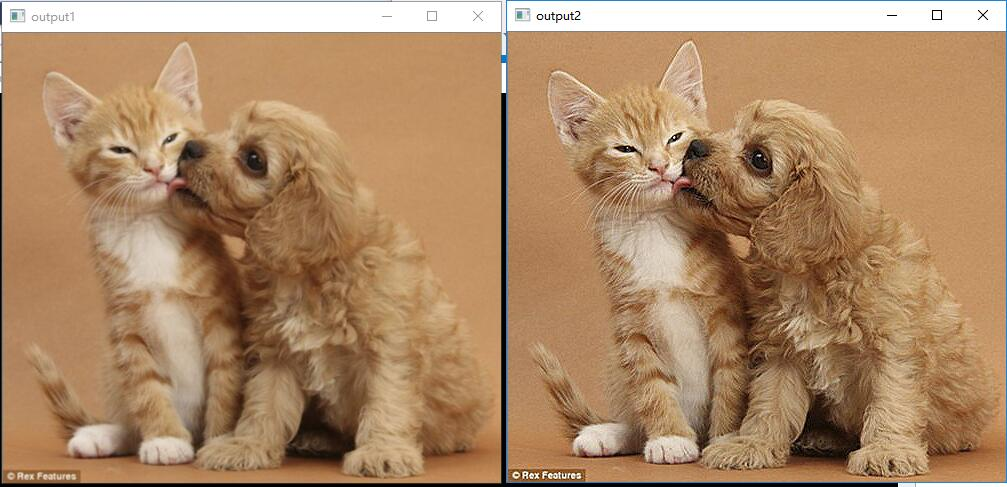# 5.测试运行时间

......
#include <time.h>
......
int main(){
clock_t start, finish;
double  duration;

start = clock();

//......
//要执行的内容
//......

finish = clock();
duration = (double)(finish - start) / CLOCKS_PER_SEC;
printf("行优先用时： %f seconds\n", duration);

return 0;
}


......
......
int main(){
double t=getTickCount();

//......
//要执行的内容
//......

double timeConsume = (getTickCount() - t) / getTickFrequency();
printf("time consume %.2f",timeConsume);

return 0;
}

###### 案例代码：
#include<opencv2/opencv.hpp>
#include<iostream>
#include<math.h>

using namespace cv;

int main(int argc,char** argv){
Mat src,dst;

if(!src.data){
return -1;
}

double t=getTickCount();

Mat kernel=(Mat_<char>(3,3)<< 0, -1, 0, -1, 5, -1 , 0, -1, 0);
filter2D(src,dst,src.depth(),kernel);
//filter2D(src,dst,-1,kernel);

double timeConsume = (getTickCount() - t) / getTickFrequency();
printf("time consume %.2f",timeConsume);

namedWindow("output1",CV_WINDOW_AUTOSIZE);
imshow("output1",src);

namedWindow("output2",CV_WINDOW_AUTOSIZE);
imshow("output2",dst);

waitKey(0);

return 0;
}


2019-04-03 10:52:25 qq_38236355 阅读数 90
• ###### MATLAB图像处理

全面系统的学习MATLAB在图像处理中的应用

19898 人正在学习 去看看 魏伟

1、获取图像像素指针

Mat.ptr(int i = 0) 获取像素矩阵的指针，索引i表示第几行，从0开始记行数。

const uchar* current = myimage.ptr<uchar>(row);


myimage.depth() = CV_8U，图像的类型为8U。

p(row,col) = current[col];


2、像素范围处理saturate_cast<uchar>

saturate_cast<uchar>（-100），返回 0。
saturate_cast<uchar>（288），返回255
saturate_cast<uchar>（100），返回100


3、掩膜操作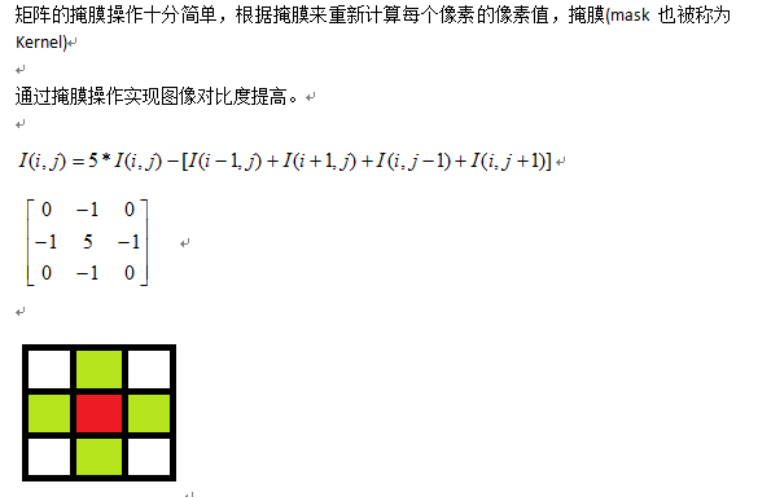4、函数调用filter2D功能

filter2D( src, dst, src.depth(), kernel );其中src与dst是Mat类型变量、src.depth表示位图深度，有32、24、8等。

5、完整代码演示

#include<opencv2\opencv.hpp>
#include<iostream>
#include<math.h>
using namespace cv;

int main(int argc, char **argv)
{
Mat src = imread("D:/opencv learning/meinv.jpg", -1);
if (src.empty())
{
return -1;
}
namedWindow("input image", WINDOW_AUTOSIZE);
imshow("input image", src);

//进行掩膜操作的代码

Mat dst = Mat::zeros(src.size(), src.type());//输出图片进行初始化。
//不调用filter 2D 函数，自己编写掩膜操作
/*
int cols = (src.cols-1) * src.channels(); //实际的长度cols等于RGB图像的长度乘以通道数。col为列，去除了最后一列
int offsetx = src.channels();//图像的通道数，例如RGB图像为3通道
int rows = (src.rows-1); //图像的高度。row为行,去除了最后一行
for (int row = 1; row < rows; row++) //从第二行到倒数第二行，去除边缘的行
{
const uchar *previous = src.ptr<uchar>(row - 1);//定义指向上一行的指针,指针是uchar型，不能是int型，会引起图像变形
const uchar *count = src.ptr<uchar>(row);//定义指向当前行的指针
const uchar *next = src.ptr<uchar>(row + 1);//定义指向下一行的指针
uchar *output = dst.ptr<uchar>(row);//定义指向输出图像当前行的指针
for (int col = offsetx; col< cols; col++)//从第二列到倒数第二列，去除边缘列
{
output[col] = saturate_cast<uchar>(5 * count[col] - (previous[col] + next[col] + count[col + offsetx] + count[col - offsetx]));
//掩膜计算公式
}
}
*/
//显示运行时间
double t = getTickCount();//当前的运行周期数

//用filter 2D 函数进行掩膜操作
Mat kernel = (Mat_<char>(3, 3) << 0, -1, 0, -1, 5, -1, 0, -1, 0);//定义掩膜
filter2D(src, dst, src.depth(), kernel);

t = ((double)getTickCount() - t) / getTickFrequency();//运行时间
std::cout << "消耗的时间：" << t << std::endl;

namedWindow("output image", WINDOW_AUTOSIZE);
imshow("output image", dst);
waitKey(0);
return 0;
}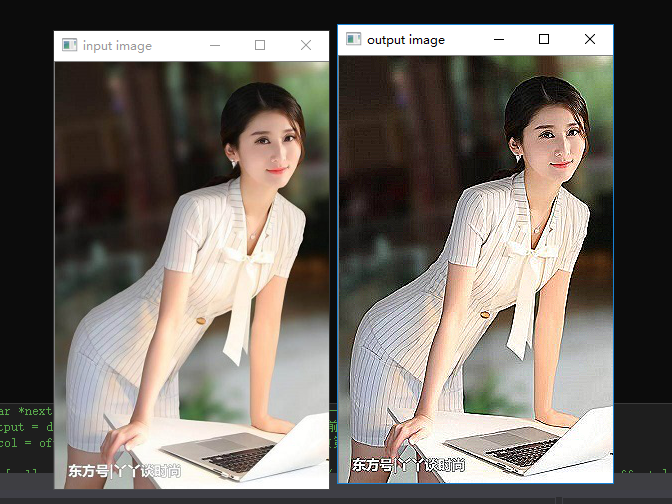2013-08-17 20:03:22 meng4411yu 阅读数 39492
• ###### MATLAB图像处理

全面系统的学习MATLAB在图像处理中的应用

19898 人正在学习 去看看 魏伟

1、掩膜的定义

掩模是由0和1组成的一个二进制图像。当在某一功能中应用掩模时，1值区域被处理，被屏蔽的0值区域不被包括在计算中。通过指定的数据值、数据范围、有限或无限值、感兴趣区和注释文件来定义图像掩模，也可以应用上述选项的任意组合作为输入来建立掩模。（来自百度百科http://baike.baidu.com/view/2062299.htm#2

2、图像掩膜例子3、百度文库还有一篇比较容易懂的关于掩膜的文档

http://wenku.baidu.com/view/bc1407d6b14e852458fb57cd.html

用选定的图像、图形或物体,对待处理的图像(全部或局部)进行遮挡,来控制图像处理的区域或处理过程。用于覆盖的特定图像或物体称为掩模或模板。光学图像处理中,掩模可以足胶片、滤光片等。数字图像处理中,掩模为二维矩阵数组,有时也用多值图像。

数字图像处理中,图像掩模主要用于：

①提取感兴趣区,用预先制作的感兴趣区掩模与待处理图像相乘,得到感兴趣区图像,感兴趣区内图像值保持不变,而区外图像值都为0。

②屏蔽作用,用掩模对图像上某些区域作屏蔽,使其不参加处理或不参加处理参数的计算,或仅对屏蔽区作处理或统计。

③结构特征提取,用相似性变量或图像匹配方法检测和提取图像中与掩模相似的结构特征。

④特殊形状图像的制作。用选定的图像、图形或物体,对待处理的图像(全部或局部)进行遮挡,来控制图像处理的区域或处理过程。用于覆盖的特定图像或物体称为掩模或模板。

4、那么我们就来看看一幅图像(傅立叶域)和掩膜相乘的结果（实验）从图2和图5，我们可以分辨出来掩膜滤掉了部分信息，只选取了掩膜“感兴趣”的区域。

2018-04-23 20:52:38 qq_21578125 阅读数 1108
• ###### MATLAB图像处理

全面系统的学习MATLAB在图像处理中的应用

19898 人正在学习 去看看 魏伟

①提取感兴趣区，用预先做好的感兴趣区掩模与待处理图像相乘，得到感兴趣区域图像，感兴趣区域内图像像素值不变，而区域外的图像像素值为0

②屏蔽作用，用掩模对图像上某些区域做屏蔽，使其不参加处理或不参与处理参数的计算，或仅对屏蔽区作处理和统计。

③结构特征提取，用相似性变量或图像匹配方法检测和提取图像中与掩模相似的结构特征。

④特殊形状图像的制作